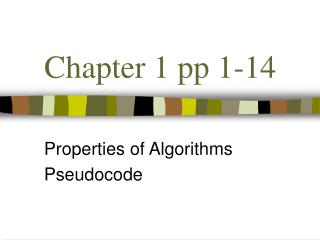# Chapter 1 pp 1-14 - PowerPoint PPT PresentationDownload PresentationChapter 1 pp 1-14

Chapter 1 pp 1-14
Download Presentation## Chapter 1 pp 1-14

- - - - - - - - - - - - - - - - - - - - - - - - - - - E N D - - - - - - - - - - - - - - - - - - - - - - - - - - -
##### Presentation Transcript

1. Chapter 1 pp 1-14 Properties of Algorithms Pseudocode

2. What is an algorithm? • You tell me.

3. Algorithms • step by step method for solving a problem. • formal solution method • implementing a problem solution with computer code is pretty darn formal

4. Algorithms have properties • Input • Output • Precision • Determinism • Finiteness • Correctness • Generality

5. Examples first • Lets look at an example first and we’ll try to identify the properties. • Max of three numbers • Shortest pair problem

6. Max of 3 numbers int Max(int a, int b, int c) { if (a > b) { if (a > c) return a; else return c; } else { if (b > c) return b; else return c; } }

7. Max of 3 • Input: 3 numbers a, b, and c • Output: 1 number that is the max of a, b, and c. (duh?) • Is the algorithm precisely defined? • What would an imprecise algorithm look like? • Code by its very nature is precise.

8. Determinism • Same input, same steps  Some outcome • Non-determinism • Same input, same steps  Different outcome • Code by its nature is deterministic • How do your write a non-deterministic program?

9. Finiteness Will it run infinitely? • The definition of an algorithm is a formally defined solution to a problem. • Is it really a solution if it runs forever?

10. Correctness • It is very easy to write incorrect code. • Verifying correctness is wicked hard • This is where proofs come in handy.

11. Generality • Can the algorithm be applied to all sets of possible input? • Here an algorithm that is correct but not general. int max(a, b) { if (a > 10 && b < 10) return a; }

12. Pseudocode • resembles C++ and Java • like short-hand max(a,b) { if a > b return a else return b } • This is where algorithms get imprecise

13. Pseudocode • easier to specify loops • easier to define data structures mystery(a[], n, x) { for i = 0 to n-1 if (x == a[i]) return true return false; }

14. Example: Shortest pair • Given n points (x,y)-pairs • Where x and y are real numbers. • Return the distance of the two closest points. • You could also return the two points • Describe how you would solve this in words.

15. #1 INPUT: Here the input is well-defined Example: Shortest pair • Given n points (x,y)-pairs • Where x and y are real numbers. • Return the distance of the two closest points. • Also return the two points • Describe how you would solve this in words. #2 OUTPUT: The details here can have big impact on the actual algorithm #3 PRECISION: Some algorithms can be precisely described with word, some cannot.

16. Example: Shortest pair • Compute the distance between every pair of points. • Return the two points with minimum distance #3 PRECISION: This is not precise because important details are not described: 1. Computing Distance is not trivial2. Iterating over every pair of points is not a simple operation.

17. Example: Shortest pair Input: An array of n points p[i] Output: A distance d, which is the minimum among all pairs of points Algorithm: d = 1000000; for i = 1 to n for j = i+1 to n temp = dist(p[i] , p[j]) if (temp < d) d = temp return d;

18. Example: Shortest pair float dist(a, b) { return sqrt((a.x – b.x)2 + (a.y – b.y)2); } Is this as precise as you can get? BTW, there are six mathematical operations here Two ( – ) one clock cycle each One ( + ) one clock cycle Two ( * ) one clock cycle each One ( sqrt function ) ? clock cycles